Homework Help Question & Answers

# 12. Use the equation midv/dt +v/t)=-eE for the electron drift velocity, v, to show that the...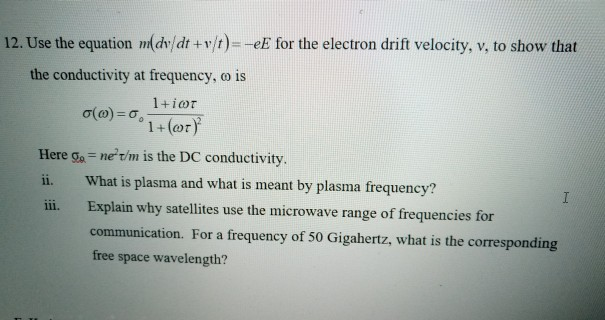12. Use the equation midv/dt +v/t)=-eE for the electron drift velocity, v, to show that the conductivity at frequency, o is (0)= 1+ior 1+(or) Here a = ne' t/m is the DC conductivity. ii. What is plasma and what is meant by plasma frequency? Explain why satellites use the microwave range of frequencies for communication. For a frequency of 50 Gigahertz, what is the corresponding free space wavelength?

#### Homework Answers

Answer #1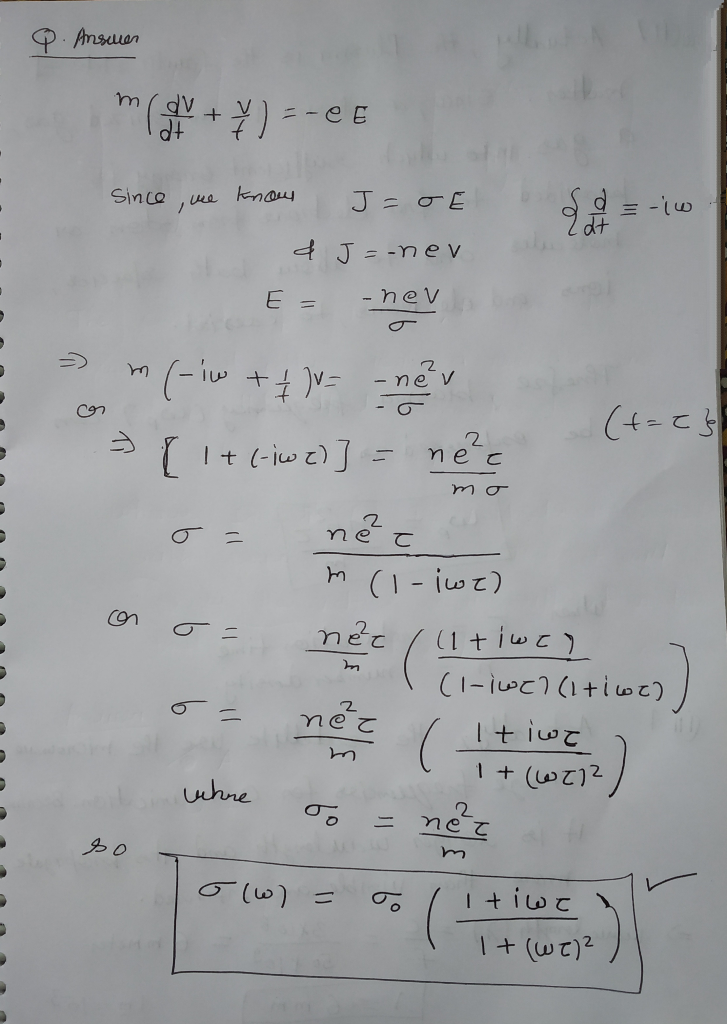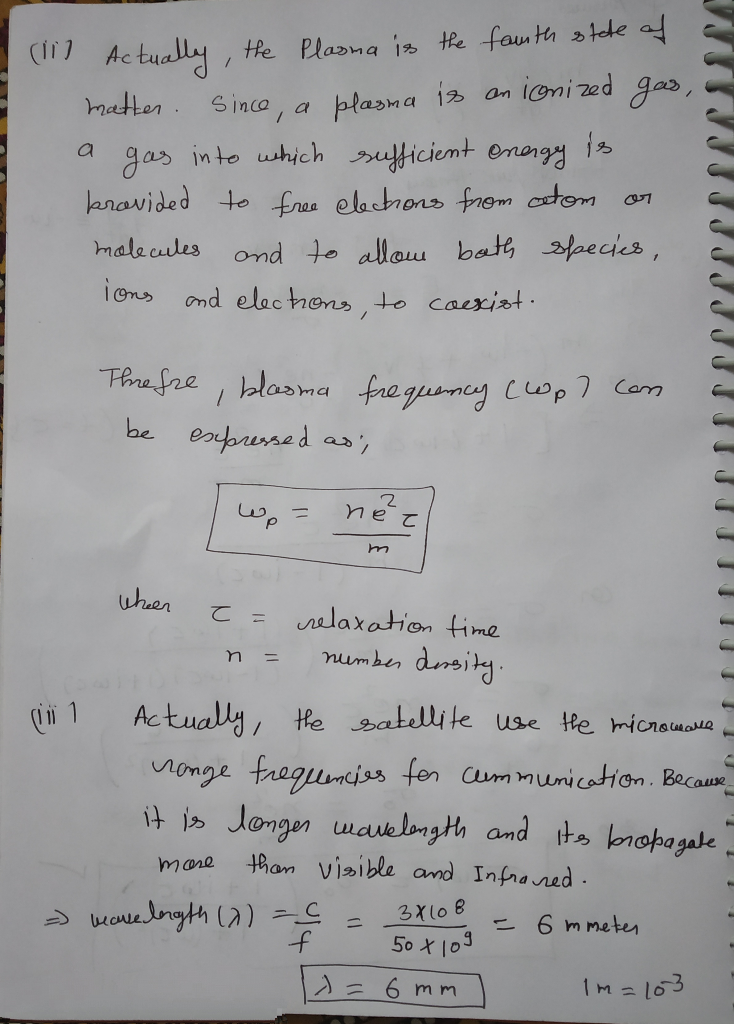please like thank you....

Know the answer?
Your Answer:

#### Post as a guest

Your Name:

What's your source?

#### Earn Coin

Coins can be redeemed for fabulous gifts.

Not the answer you're looking for? Ask your own homework help question. Our experts will answer your question WITHIN MINUTES for Free.
Similar Homework Help Questions
• ### 6. Frequency dependence of the electrical conductioily. Use the equation midoldt +uh)--eE for the electron drift...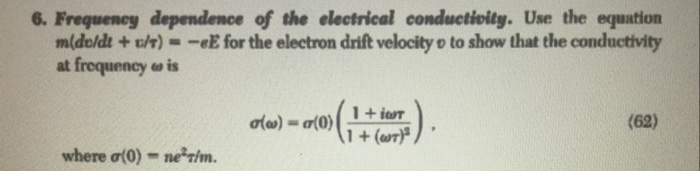6. Frequency dependence of the electrical conductioily. Use the equation midoldt +uh)--eE for the electron drift velocity o to show that the conductivity at frequency e is (62) + Gar where ơ(0)-nerin.

• ### es anh th e c dfe sod aao rt34e k-e: e fi、c ︵ee aod g ti r mdfdpe hrr nd s pt Ide r. csa reu etnn doi ers inas dav si a)rec.z trlar k-te eer 0 dkne nro li ob "bee tdgu sa 9 edeb onfr fr cfr pge...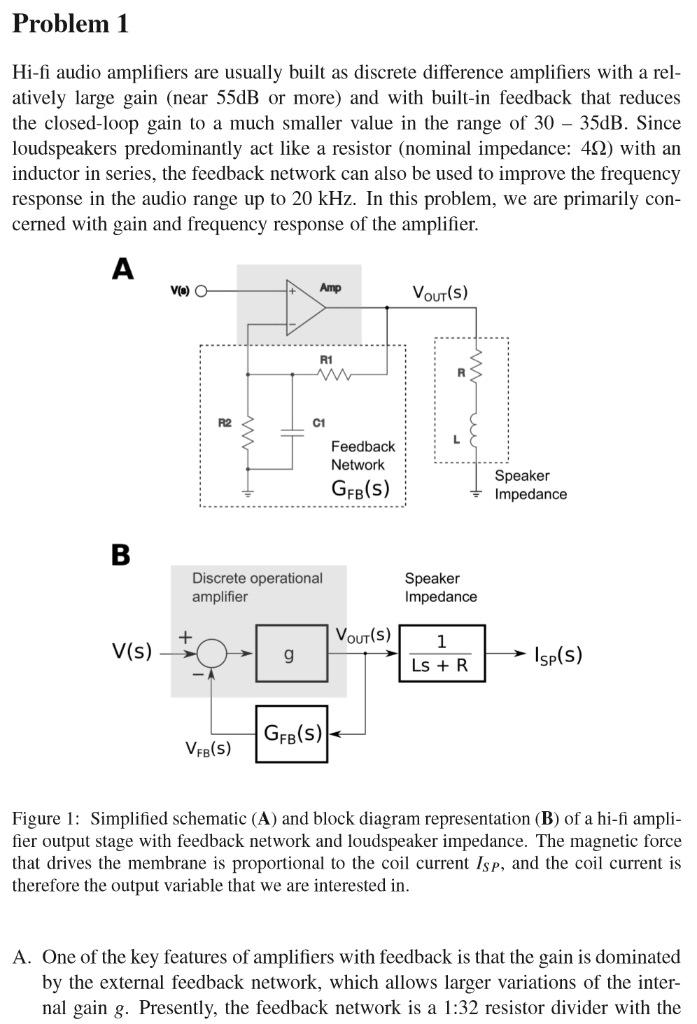es anh th e c dfe sod aao rt34e k-e: e fi、c ︵ee aod g ti r mdfdpe hrr nd s pt Ide r. csa reu etnn doi ers inas dav si a)rec.z trlar k-te eer 0 dkne nro li ob "bee tdgu sa 9 edeb onfr fr cfr pgeo etg 0 uet tio edl tnd gra .1.1 euds P Hall in re ce capacitor C1 omitted. Therefore, VFB(t) VouT)/32. We assume g -800 What is the actual gain of...

• ### 1. The magnetic field of a wave propagating through a certain nonmagnetic material is given by...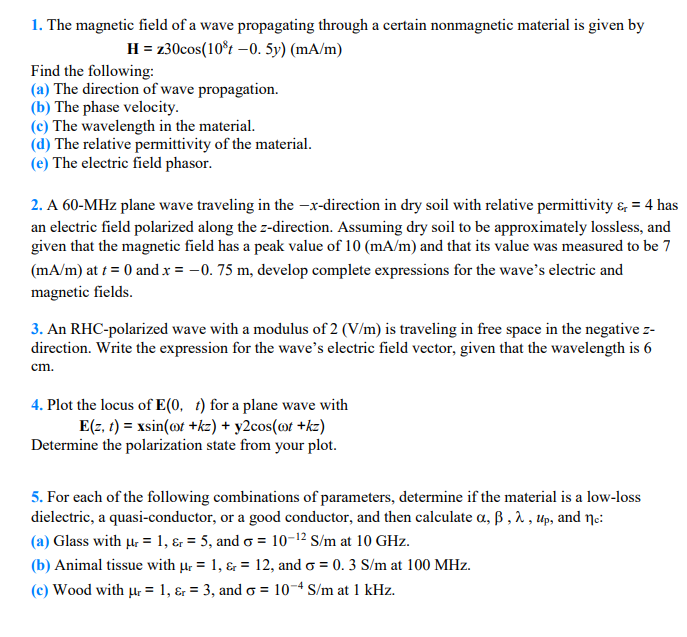1. The magnetic field of a wave propagating through a certain nonmagnetic material is given by H-x30cos(10%-0. Sy) (mA/m) Find the following: (a) The direction of wave propagation. (b) The phase velocity (c) The wavelength in the material. (d) The relative permittivity of the material (e) The electric field phasor 2. A 60-MHz plane wave traveling in the -x-direction in dry soil with relative permittivity &4 has an electric field polarized along the z-direction. Assuming dry soil to be approximately...

Free Homework App

Scan Your Homework
to Get Instant Free Answers
Need Online Homework Help?

Get Answers For Free
Most questions answered within 3 hours.